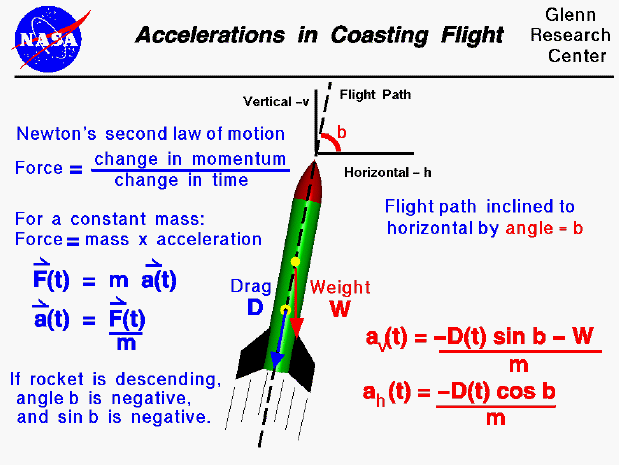The forces on a model rocket change dramatically in both magnitude and direction throughout a typical flight. This figure shows the accelerations on a rocket during the coasting portion of the flight following powered flight. The acceleration is produced in response to Newton's first law of motion. During most of the coasting flight a net external force is being applied to the rocket. During coasting flight, the rocket engine is no longer producing thrust, so the only forces acting on the rocket are weight and drag.

The acceleration (or deceleration) of a coasting model rocket is described by Newton's second law of motion. In general, Newton's law prescribes that the force on an object (F) is equal to the change in momentum (mass times velocity) per unit time. Since the mass (m) is constant (no fuel is being burned), the equation becomes the more familiar force equals mass times acceleration, ( F = m * a ). The force and acceleration are vector quantities having both a magnitude and a direction while the mass is a scalar and has only a magnitude. (Mathematicians will often denote a vector with a small arrow over the symbol). For the model rocket, the drag (D) force changes with time (t). To denote that drag, and therefore the acceleration, is a function of time we include the "(t)" symbol next to this variable. Newton's second law is then written as [ F(t) = m * a(t) ]. We can use algebra to solve for the accelerations: [ a(t) = F(t) / m ].

Under ideal conditions, the flight path of the model rocket would be perfectly vertical. In practice, the flight path is inclined due to the action of the wind. The angle which the flight path makes with the horizontal is called the inclination angle (b). If we consider the forces in the vertical (v) and horizontal (h) directions, we obtain two equations for the acceleration; one for the vertical and one for the horizontal. The "cos b" and "sin b" are the trigonometric functions cosine and sine of the inclination angle. The vertical acceleration is given by the following equation: ( av(t) = ( - D(t) * sin b - W ) / m ). The horizontal acceleration is give by: ( ah(t) = ( - D(t) * cos b ) / m ). If the flight path was perfectly vertical, the inclination angle would be 90 degrees. The cosine of 90 degrees is zero, so the horizontal acceleration would be zero. The sine of 90 degrees is 1.0, so the vertical deceleration (negative acceleration) would equal the drag plus the weight divided by the mass. Since there is no force in the positive vertical direction, an upward coasting vehicle is always slowing down until a point is reached when the vertical velocity is zero. The drag then becomes zero, but the weight is still acting on the rocket and it will begin to fall back to earth. The inclination angle and the sine of the inclination angle are negative during the descent. Considering the vertical acceleration equation, the drag and weight are in opposition during descent (the drag term is positive and the weight is negative). The rocket gains speed as it falls. Since the speed increases, the drag increases and a point is soon reached where the drag is equal and opposite to the weight. Under these conditions, the rocket vertical acceleration is zero and the rocket falls at a constant terminal velocity.